Integrating of fuzzy Shannon's entropy with fuzzy TOPSIS for industrial robotic system section

Integration of Fuzzy Shannon’s Entropy with Fuzzy TOPSIS for industrial robotic system selection

University of Tehran, (IRAN)

Accepted February 2012

Jafarnejad Chaghooshi, A. Fathi, M.R., & Kashef, M. (2012). Integration of Fuzzy Shannon’s Entropy with Fuzzy TOPSIS for industrial robotic system selection. Journal of Industrial Engineering and Management, 5(1), 102-114. http://dx.doi.org/10.3926/jiem.397

---------------------

Abstract:

Purpose: The aim of this study is applying a new method for Industrial robotic system selection.

Design/methodology/approach: In this paper, the weights of each criterion are calculated using fuzzy Shannon’s Entropy. After that, fuzzy TOPSIS is utilized to rank the alternatives. After that we compare the result of Fuzzy TOPSIS with Fuzzy VIKOR method. Then we select the best Industrial Robotic System based on these results.

Findings: The outcome of this research is ranking and selecting industrial robotic systems with the help of Fuzzy Shannon’s Entropy and Fuzzy TOPSIS techniques.

Originality/value: This paper offers a new integrated method for industrial robotic system selection.

Keywords: entropy, TOPSIS, fuzzy set, robotic systems

---------------------

1 Introduction

Recent developments in information technology and engineering sciences have been the main reason for the increased utilization of robots in a variety of advanced manufacturing facilities. Robots with vastly different capabilities and specifications are available for a wide range of applications (Rao, 2007). The selection of robots to suit a particular application and production environment from among the large number available in the market has become a difficult task. Various aspects such as product design, production system, and economics, need to be considered before a suitable robot can be selected. The selection problem is particularly relevant in view of the likely lack of experience of prospective users in employing a robot. Indeed, robots are still a new concept in industry as a whole, and so it is not unusual for an industry to be a first-time robot purchaser (Rao, 2007). Many precision-based methods for robot selection have been developed to date. Boubekri, Sahoui and Lakrib (1991) developed an expert system for industrial robot selection considering functional, organizational, and economical attributes in the selection process. Wang, Singh and Huang (1991) presented a decision support system that applies a fuzzy set method for robot selection. The objective attributes were evaluated via marginal value functions while the subjective attributes were evaluated via fuzzy set membership function. Data from both evaluations were finally processed such that a fuzzy set decision vector was obtained. However, the fuzzy method presented is a complicated one, and requires more computation. Booth, Khouja and Hu (1992) proposed a decision model for the robot selection problem using both Mahalanobis distance analysis, i.e., a multivariate distance measure, and principal-components analysis. Liang and Wang (1993) proposed a robot selection algorithm by combing the concepts of fuzzy set theory and hierarchical structure analysis. The algorithm was used to aggregate decision makers’ fuzzy assessments about robot selection attributes weightings, and to obtain fuzzy suitability indices. The suitability ratings were then ranked to select the most suitable robot. Khouja and Offodile (1994) reviewed the literature on industrial robots selection problems and provided directions for future research. Khouja (1995) presented a two-phase robot selection model that involved the application of data envelopment analysis (DEA) in the first phase, and a multi-attribute decision-making model in the second phase. The rest of the paper is organized as follows: The following section presents a concise treatment of the basic concepts of fuzzy set theory. Section 3 presents the methodology. The application of the proposed method is addressed in Section 4. Finally, conclusions are provided in Section 5.

2 Fuzzy sets and fuzzy numbers

Fuzzy set theory, which was introduced by Zadeh (1965) to deal with problems in which a source of vagueness is involved, has been utilized for incorporating imprecise data into the decision framework. A fuzzy set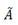can be defined mathematically by a membership function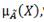which assigns each element x in the universe of discourse X a real number in the interval [0,1]. A triangular fuzzy numbercan be defined by a triplet (a, b, c) as illustrated in Figure 1.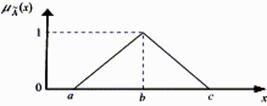Fig 1. A triangular fuzzy numberThe membership function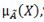is defined as(1)

Basic arithmetic operations on triangular fuzzy numbers A1 = (a1,b1,c1), where  a1 ≤ b1 ≤ c1, and A2 = (a2,b2,c2), where a2 ≤ b2 ≤ c2,can be shown as follows:(2)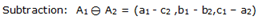(3)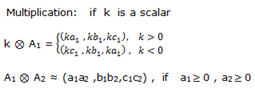(4)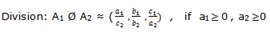(5)

Although multiplication and division operations on triangular fuzzy numbers do not necessarily yield a triangular fuzzy number, triangular fuzzy number approximations can be used for many practical applications (Kaufmann & Gupta, 1988). Triangular fuzzy numbers are appropriate for quantifying the vague information about most decision problems including personnel selection (e.g. rating for creativity, personality, leadership, etc.). The primary reason for using triangular fuzzy numbers can be stated as their intuitive and computational-efficient representation (Karsak, 2002). A linguistic variable is defined as a variable whose values are not numbers, but words or sentences in natural or artificial language. The concept of a linguistic variable appears as a useful means for providing approximate characterization of phenomena that are too complex or ill-defined to be described in conventional quantitative terms (Zadeh, 1975).

3 Research methodology

In this paper, the weights of each criterion are calculated using fuzzy Shannon’s Entropy. After that, fuzzy TOPSIS is utilized to rank the alternatives. In this paper, Fuzzy VIKOR method is used to compare the result of Fuzzy TOPSIS. Finally, we select the best Industrial Robotic System based on these results.

3.1 The fuzzy TOPSIS method

TOPSIS views a MADM problem with m alternatives as a geometric system with m points in the n-dimensional space. The method is based on the concept that the chosen alternative should have the shortest distance from the positive-ideal solution and the longest distance from the negative-ideal solution. TOPSIS defines an index called similarity to the positive-ideal solution and the remoteness from the negative-ideal solution. Then the method chooses an alternative with the maximum similarity to the positive-ideal solution (Wang & Chang, 2007). It is often difficult for a decision-maker to assign a precise performance rating to an alternative for the attributes under consideration. The merit of using a fuzzy approach is to assign the relative importance of attributes using fuzzy numbers instead of precise numbers. This section extends the TOPSIS to the fuzzy environment (Yang & Hung, 2007). This method is particularly suitable for solving the group decision-making problem under fuzzy environment. We briefly review the rationale of fuzzy theory before the development of fuzzy TOPSIS. The mathematics concept borrowed from Ashtiani, Haghighirad, Makui and Montazer (2009), (Büyüközkan, Feyziog-Lu & Nebol, 2007) and (Wang & Chang, 2007).

Step 1: Determine the weighting of evaluation criteria

A systematic approach to extend the TOPSIS is proposed to selecting an Industrial Robotic System under a fuzzy environment in this section. In order to perform a pairwise comparison among the parameters, a linguistic scale has been developed. Our scale is depicted in Figure 2 and the corresponding explanations are provided in Table 1. We have used five main linguistic terms to compare the criteria: ‘‘equal importance’’, ‘‘moderate importance’’, ‘‘strong importance’’, ‘‘very strong importance’’ and ‘‘demonstrated importance’’. We have also considered their reciprocals: ‘‘equal unimportance’’, ‘‘moderate unimportance’’, ‘‘strong unimportance’’, ‘‘very strong unimportance’’ and ‘‘demonstrated unimportance’’. For instance, if criterion A is evaluated ‘‘strongly important’’ than criterion B, then this answer means that criterion B is ‘‘strongly unimportant’’ than criterion A.Figure 2. Membership functions of triangular fuzzy numbers corresponding to the linguistic scale

 Linguistic scale Triangular fuzzy numbers The inverse of triangular fuzzy numbers Equal Importance (1, 1, 1) (1, 1, 1) Moderate Importance (1, 3, 5) (1/5, 1/3, 1) Strong importance (3, 5, 7) (1/7, 1/5, 1/3) Very strong importance (5, 7, 9) (1/9, 1/7, 1/5) Demonstrated importance (7, 9, 11) (1/11, 1/9, 1/7)

Table 1. The linguistic scale and corresponding triangular fuzzy numbers

Step 2: Construct the fuzzy decision matrix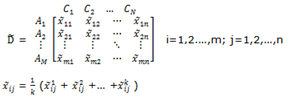(6)

Where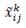is the rating of alternative Ai with respect to criterion Cj evaluated by expert and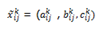Step 3: Normalize the fuzzy decision matrix

The normalized fuzzy decision matrix denoted by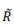is shown as following formula: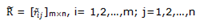(7)

Then the normalization process can be performed by following formula:

Where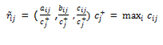The normalized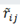are still triangular fuzzy numbers. For trapezoidal fuzzy numbers, the normalization process can be conducted in the same way. The weighted fuzzy normalized decision matrix is shown as following matrix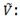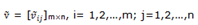(8)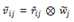(9)

Step 4: Determine the fuzzy positive-ideal solution (FPIS) and fuzzy negative-ideal solution (FNIS)

According to the weighted normalized fuzzy decision matrix, we know that the elements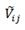are normalized positive TFNs and their ranges belong to the closed interval [0, 1]. Then, we can define the FPIS A+ and FNIS A- as following formula:(10) (11)

Step 5: Calculate the distance of each alternative from FPIS and FNIS

The distances (di+ and di-) of each alternative A+ from and A- can be currently calculated by the area compensation method.(12) (13)

Step 6: Obtain the closeness coefficient and rank the order of alternatives

The CCi is defined to determine the ranking order of all alternatives once the di+ and di- of each alternative have been calculated. Calculate similarities to ideal solution. This step solves the similarities to an ideal solution by formula: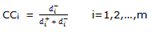(14)

According to the CCi, we can determine the ranking order of all alternatives and select the best one from among a set of feasible alternatives.

3.2 Fuzzy Shannon’s entropy based on a- level sets

Hosseinzadeh, Lotfi and Fallahnejad (2010) extend the Shannon entropy for the imprecise data, especially interval and fuzzy data cases. In this paper we obtain the weights of criteria based on their method. The steps of fuzzy Shannon’s Entropy explained as follow (Hosseinzadeh et al., 2010):

Step 1: Transforming fuzzy data into interval data by using the a-level sets:

The a-level set of a fuzzy variableis defined by a set of elements that belong to the fuzzy variablewith membership of at least a i.e.,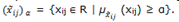The a-level set can also be expressed in the following interval form: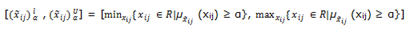(15)

where 0 < a ≤ 1. By setting different levels of confidence, namely 1-a, fuzzy data are accordingly transformed into different a-level sets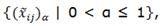which are all intervals.

Step 2: The normalized values pij  and pij’’ are calculated as: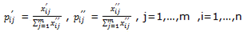(16)

Step 3: Lower bound hi and upper bound hi’’ of interval entropy can be obtained by:(17)

where h0 is equal to (Ln m)-1, and pij.Ln pij or pij’’.Ln pij’’ is defined as 0 if pij= 0 or pij’’= 0.

Step 4: Set the lower and the upper bound of the interval of diversification di and di’’ as the degree of diversification as follows: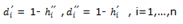(18)

Step 5: Set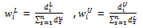, i=1,…,n as the lower and upper bound of interval weight of attribute i.

4 Numerical example

In this section, we demonstrate the application of this method by numerical example. Through the literature investigation and studying other papers that are related to robotic system selection, finally ten criteria are selected. These criteria include purchasing cost (C1), Maintenance Cost (C2), Training Cost (C3), Labor Cost (C4), Repeatability error (C5), Speed (C6), Load carrying capacity (C7), Man-machine interface (C8), Memory capacity (C9) and accuracy (C10). In addition, there are four alternatives include A1, A2, A3 and A4.

4.1 Fuzzy Shannon’s Entropy

In fuzzy Shannon’s Entropy, firstly, the criteria and alternatives’ importance weights must be compared. Afterwards, the comparisons about the criteria and alternatives, and the weight calculation need to be made. Thus, the evaluation of the criteria according to the main goal and the evaluation of the alternatives for these criteria must be realized. Then, after all these evaluation procedure, the weights of the alternatives can be calculated. In the second step, these weights are used to Fuzzy TOPSIS calculation for the final evaluation. The aggregate decision matrix for Shannon’s Entropy can be seen from Table 2.

 DM C1 C2 C3 … C10 A1 (0.75, 1.00,1.00) (0.75, 1.00,1.00) (0.75, 1.00,1.00) … (0.75, 1.00,1.00) A2 (0.25, 0.50,0.75) (0.00, 0.00,0.25) (0.25, 0.50,0.75) … (0.25, 0.50,0.75) A3 (0.00, 0.25,0.50) (0.25, 0.50,0.75) (0.50, 0.75,1.00) … (0.50, 0.75,1.00) A4 (0.25, 0.50,0.75) (0.50, 0.75,1.00) (0.50, 0.75,1.00) … (0.00, 0.00,10.25)

Table 2. Aggregate decision matrix for fuzzy Shannon’s Entropy

After forming decision matrix, we transformed fuzzy data of Table 2 into interval data. For transforming fuzzy data into interval data, we consider a = 0.3. The interval decision matrix can be seen from Table 3.

 DM C1 C2 C3 … C10 A1 [0.82,1.00] [0.82,1.00] [0.82,1.00] … [0.82,1.00] A2 [0.32,0.67] [0.00,0.17] [0.32,0.67] … [0.32,0.67] A3 [0.07,0.42] [0.32,0.67] [0.57,0.92] … [0.57,0.92] A4 [0.32,0.67] [0.57,0.92] [0.57,0.92] … [0.00,0.17]

Table 3. Interval decision matrix

Then, according to Eq. (16), we normalized the interval decision matrix. The normalized interval decision matrix is shown in Table 4.

 DM C1 C2 C3 … C10 A1 [0.029,0.645] [0.297,0.579] [0.234,0.434] … [0.297,0.579] A2 [0.117,0.435] [0.000,0.101] [0.092,0.293] … [0.117,0.391] A3 [0.027,0.274] [0.117,0.391] [0.163,0.402] … [0.207,0.536] A4 [0.117,0.435] [0.207,0.536] [0.163,0.402] … [0.000,0.101]

Table 4. The normalized interval decision matrix

In the next step, we calculate the lower bound hi and upper bound hi’’ of criteria based on the Eq. (17). After that the degrees of diversification are calculated using Equation (18), as shown in Table 5.

 [hi’, hi’’] [di’, di’’] C1 [0.41,0.59] [0.40,0.58] C2 [0.40,0.54] [0.45,0.59] C3 [0.49,0.63] [0.36,0.50] C4 [0.35,0.48] [0.51,0.64] C5 [0.38,0.56] [0.43,0.61] C6 [0.42,0.55] [0.44,0.57] C7 [0.40,0.55] [0.44,0.59] C8 [0.51,0.63] [0.36,0.48] C9 [0.26,0.28] [0.71,0.73] C10 [0.40,0.54] [0.45,0.59]

Table 5. The values of hi, hi’’, di and di’’

Finally, the interval weight and crisp weight are calculated, as shown in Table 6.

 [wiL, wiU] Wi C1 [0.088,0.098] 0.0936 C2 [0.099,0.100] 0.0997 C3 [0.079,0.084] 0.0822 C4 [0.108,0.110] 0.1097 C5 [0.094,0.104] 0.0993 C6 [0.095,0.097] 0.0970 C7 [0.095,0.100] 0.0978 C8 [0.079,0.082] 0.0810 C9 [0.124,0.154] 0.1396 C10 [0.099,0.100] 0.0997

Table 6. The interval and crisp weight of criteria

4.2 Fuzzy TOPSIS

The weights of the alternatives are calculated by fuzzy Shannon’s Entropy up to now, and then these values can be used in fuzzy TOPSIS. Thus, normalized decision matrix can be prepared. This matrix can be seen from Table 7.

 C1 C2 C3 … C10 A1 (0.75, 1.00,1.00) (0.75, 1.00,1.00) (0.75, 1.00,1.00) … (0.75, 1.00,1.00) A2 (0.50, 0.75,0.00) (0.00, 0.00,0.25) (0.25, 0.50,0.75) … (0.25, 0.50,0.75) A3 (0.00, 0.25,0.50) (0.25, 0.50,0.75) (0.50, 0.75,1.00) … (0.50, 0.75,1.00) A4 (0.25, 0.50,0.75) (0.50, 0.75,1.00) (0.50, 0.75,1.00) … (0.00, 0.00,0.25) Wj 0.0936 0.0997 0.0822 … 0.0977

Table 7. The normalized decision matrix

By following fuzzy TOPSIS procedure steps and calculations, the ranking of Industrial robotic systems are gained. The results and final ranking are shown in Table 8.

 d+ d- CC Rank A1 0.01915 0.83314 0.98 2 A2 0.01330 0.74218 0.98 1 A3 0.02288 0.75283 0.97 3 A4 0.02145 0.57602 0.96 4

Table 8. Final evaluation of the alternatives

The fuzzy TOPSIS results are shown in Table 8. The evaluation of Industrial robotic systems is realized and according to the CCi values the ranking of robotic systems are A2 – A1 – A3 – A4 from most preferable to least. If the best one is needed to be selected, then the alternative A2 must be chosen. After that we ranked Industrial robotic systems based on fuzzy VIKOR procedure. The results of Fuzzy VIKOR and Fuzzy TOPSIS are shown in Table 9. According to Fuzzy VIKOR method, A3 is the best alternative that should be chosen.

 Ranking by FTOPSIS Ranking by FVIKOR A1 2 3 A2 1 2 A3 3 1 A4 4 4

Table 9. Ranking by Fuzzy VIKOR and Fuzzy TOPSIS Methods

5 Conclusion

Industrial robots have been increasingly used by many manufacturing firms in different industries. Although the number of robot manufacturers is also increasing with many alternative ranges of robots, potential end users are faced with many factors in the evaluation of the industrial robotic systems. A two-step fuzzy Shannon’s Entropy and fuzzy TOPSIS methodology is structured here that fuzzy TOPSIS uses fuzzy Shannon’s Entropy result weights as input weights. Then a numerical example is presented to show applicability and performance of the methodology. After that the results of fuzzy TOPSIS compare with Fuzzy VIKOR method. It can be said that using linguistic variables makes the evaluation process more realistic. Because evaluation is not an exact process and has fuzziness in its body. Here, the usage of fuzzy Shannon’s Entropy weights in fuzzy TOPSIS makes the application more realistic and reliable. It is clear that the selection of an industrial robotic system is a difficult. However, the developed fuzzy method seems to be usable for the solution of this problem.

References

Ashtiani, B., Haghighirad, F., Makui, A., & Montazer, G. A. (2009).Extension of fuzzy TOPSIS method based on interval-valued fuzzy sets. Applied Soft Computing, 9(2), 457-461. http://dx.doi.org/10.1016/j.asoc.2008.05.005

Booth, D. E., Khouja, M., & Hu, M. (1992).A robust multivariate statistical procedure for evaluation and selection of industrial robots.International Journal of Operations & Production Management, 12, 15–24. http://dx.doi.org/10.1108/01443579210009023

Boubekri, N., Sahoui, M., & Lakrib, C. (1991).Development of an expert system for industrial robot selection.Computers & Industrial Engineering, 20, 119–127. http://dx.doi.org/10.1016/0360-8352(91)90047-A

Büyükozkan, G., Feyziog-lu, O., & Nebol, E. (2007).Selection of the strategic alliance partner in logistics value chain. International Journal of Production Economics, 113(1), 148–158.

Hosseinzadeh Lotfi, F., & Fallahnejad, R. (2010). Imprecise Shannon’s entropy and multi attribute decision making. Entropy, 12, 53-62.  http://dx.doi.org/10.3390/e12010053

Karsak, E. E. (2002). Distance-based fuzzy MCDM approach for evaluating flexible manufacturing system alternatives.International Journal of Production Research, 40(13), 3167–3181. http://dx.doi.org/10.1080/00207540210146062

Kaufmann, A., & Gupta, M. M. (1988).Fuzzy mathematical models in engineering and management science. Amsterdam: North-Holland.

Khouja, M. (1995).The use of data envelopment analysis for technology selection.Computers & Industrial Engineering, 28, 123–132. http://dx.doi.org/10.1016/0360-8352(94)00032-I

Khouja, M., & Offodile, O. F. (1994). The industrial robots selection problem: A literature review and directions for future research. IIE Transactions, 26, 50–61. http://dx.doi.org/10.1080/07408179408966618

Liang, G. H., Wang, M. J. (1993).A fuzzy multi-criteria decision-making approach for robot selection. Robotics and Computer Aided Manufacturing, 10, 267–274. http://dx.doi.org/10.1016/0736-5845(93)90040-Q

Rao, R. V. (2007). Decision making in the manufacturing environment: using graph theory and fuzzy multiple attribute decision making methods. London: Springer.

Wang, M. J., Singh, H. P., & Huang, W. V. (1991).A decision support system for robot selection. Decision Support Systems, 7, 273–283. http://dx.doi.org/10.1016/0167-9236(91)90044-C

Wang, T. C., & Chang, T. H. (2007). Application of TOPSIS in evaluating initial training aircraft under a fuzzy environment. Expert Systems with Applications, 33, 870–880. http://dx.doi.org/10.1016/j.eswa.2006.07.003

Yang, T., & Hung, C. C. (2007). Multiple-attribute decision making methods for plant layout design problem. Robotics and Computer-Integrated Manufacturing, 23(1), 126–137. http://dx.doi.org/10.1016/j.rcim.2005.12.002

Zadeh, L. A. (1965). Fuzzy sets. Information and Control, 8(3), 338–353. http://dx.doi.org/10.1016/S0019-9958(65)90241-X

Zadeh, L. A. (1975). The concept of a linguistic variable and its application to approximate reasoning-I. Information Sciences, 8(3), 199–249. http://dx.doi.org/10.1016/0020-0255(75)90036-5

Journal of Industrial Engineering and Management, 2012

Article's contents are provided on a Attribution-Non Commercial 3.0 Creative commons license. Readers are allowed to copy, distribute and communicate article's contents, provided the author's and Journal of Industrial Engineering and Management's names are included. It must not be used for commercial purposes. To see the complete license contents, please visit http://creativecommons.org/licenses/by-nc/3.0/.